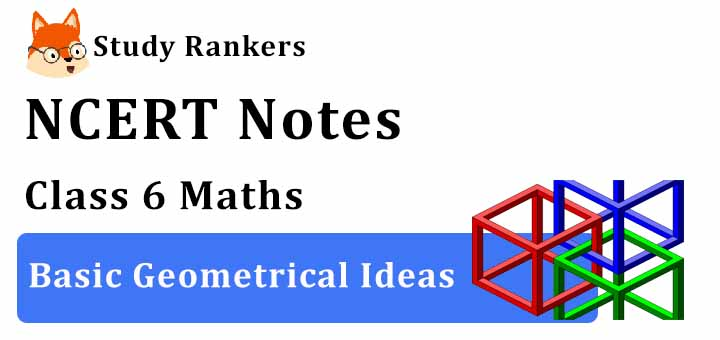# Chapter 4 Basic Geometrical Ideas Class 6 Notes Maths

Given Chapter 4 Basic Geometrical Ideas Class 6 Notes Maths will let you know important concepts embedded in the chapter in a precise manner. This make easier for the students to comprehend the concepts due to use of easy language. Class 6 Maths Revision Notes will help the students to recall information with more precision and faster. Also, NCERT Solutions for Class 6 Chapter 6 Maths that will serve as beneficial tool that can be used to recall various questions any time. It will allow the students to evaluate their learning immediately.Introduction

• The term ‘Geometry’ is the English equivalent of the Greek word ‘Geometron’. ‘Geo’ means Earth and ‘metron’ means Measurement.

Point

• A point determines a location and has no length, breadth and height. Usually denoted by a single capital letter like A, B, C.

Line Segment

• A part of a line is called a line segment. It is the shortest distance between two points.

A Line

• A line is a straight one- dimensional figure that has no thickness and extends endlessly in both directions.

Intersecting Lines

• If two lines have one common point, they are called intersecting lines.

Parallel Lines

• Two parallel lines in a plane do not meet each other.

• The perpendicular distance between two parallel lines is the same throughout.

Curves

• Any drawing (straight or non straight) done without lifting the pencil. This means, in mathematics, a line is also a curve.

• A simple curve is one that does not cross itself.

• A curve is said to be closed if its ends are joined.

• A curve is said to be open if its ends are open.

• Polygon is a simple closed curve made up of line segments. Sides: The line segment forming a polygon.

• Vertex: The meeting point of a pair of sides.

• Adjacent sides: Any two sides with a common end point.

• Adjacent vertices: The end points of the same side of a polygon.

• Diagonal: The line joining any two non-adjacent vertices.

Angles

• An angle is made up of two rays starting from a common initial point.

• The two rays forming the angle are called the arms or sides of the angle.

• The common initial point is the vertex of the angle.

• A triangle is a three-sided polygon.

• A four sided polygon is a quadrilateral. It has 4 sides and 4 angles.

Circles

• A circle is a simple closed curve which is not a polygon.

• The path of a point moving at the same distance from a fixed point. The fixed point is the centre.

• Radius: A line segment that connects the centre to a point on the circle.

• Diameter: A line segment passing through the centre and having its end points on the circle.

• Chord: A line segment connecting any two points on a circle.

• A diameter is a chord passing through the centre of a circle. It is a longest chord of a circle.

• Arc: A portion of a circle.

• Sector: A region in the interior of a circle enclosed by an arc on one side and a pair of radii on the other two sides.

• Segment: A region in the interior of a circle enclosed by an arc and chord.

• Circumference: The distance around a circle.

• Semi-circle: A diameter of a circle divides it into two equal parts, each part is a semi-circle.